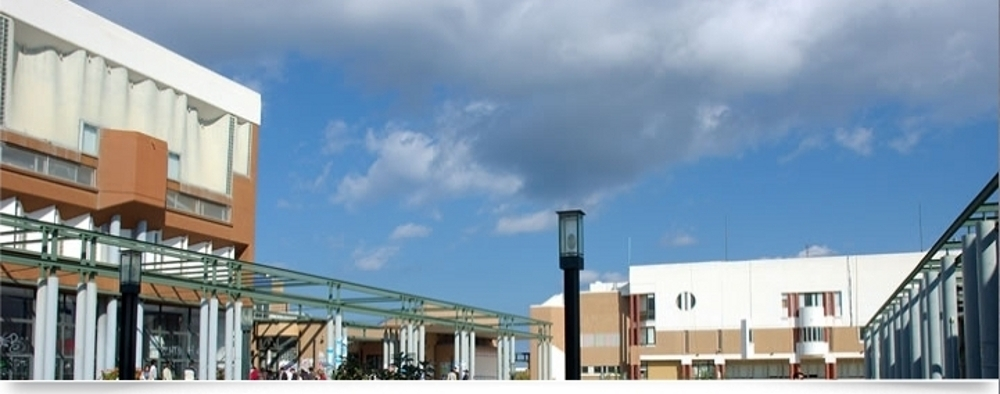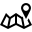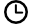# Κατάλογος Εκδηλώσεων

12
Φεβ

Ομιλία Καθηγητή Διονύσιου Χριστόπουλου
Κατηγορία: Ομιλία/ΔιάλεξηΛ - Κτίριο Επιστημών/ΗΜΜΥ, 141Π-98,141Θ-97, Αμφιθέατρο Κτιρίου Επιστημών12/02/2020 13:15 - 16:00

Περιγραφή:

Sparse Models for Space-Time Environmental Data Inspired from Statistical Physics

Dionissios T. Hristopulos
Geostatistics Laboratory, School of Mineral Resources Engineering
Technical University of Crete - Chania 73100, Greece
E-mail: dionisi@mred.tuc.gr
Laboratory Web: http://www.geostatistics.tuc.gr/index.php?id=4908

Abstract

This presentation will focus on models for space-time data based on statistical physics. Statistical physics provides a general framework for developing space-time models using Boltzmann-Gibbs probability density functions and stochastic partial differential equations (SPDEs). In geostatistics and in machine learning, spatial models are typically defined in terms of an explicit covariance (kernel) function. In contrast, in the Boltzmann-Gibbs approach the covariance function is intrinsically generated from the underlying joint probability density model. The latter is determined from a respective energy function which incorporates interactions between different locations and times. In the SPDE formulation, the covariance function is determined from the stochastic equation of motion of the random field, which leads to a respective partial differential equation for the covariance function.

I will briefly discuss the connection between the Boltzmann-Gibbs and SPDE formulations for Gaussian random fields. I will then review some results which are based on Boltzmann-Gibbs densities equipped with an energy function that comprises short-range interactions. These results include: (1) A class of flexible spatial covariance functions; (2) a non-separable covariance function with a composite space-time metric; (3) a family of non-separable covariance functions that are based on linear response theory combined with the space transform; and (4) ongoing efforts to generalize Boltzmann-Gibbs models from continuum and lattice spaces to irregular sampling geometries. The space-time models generated by means of the Boltzmann-Gibbs formulation with short-range energy functions involve by construction sparse precision matrices.  This is a significant advantage for the processing of big spatial or space-time datasets, since the computationally demanding inversion of large covariance matrices (which is common in geostatistics and Gaussian process regression) is avoided. I will illustrate these concepts with applications to environmental and energy resources datasets.

References

1. D. T. Hristopulos and S. N. Elogne, 2007. Analytic properties and covariance functions for a new class of generalized Gibbs random fields. IEEE Transactions on Information Theory, 53(12):4667–4679.
2. D. T. Hristopulos. Stochastic Local Interaction (SLI) model: Bridging machine learning and geostatistics. Computers and Geosciences, 85(Part B):26–37, 2015.
3. D. T. Hristopulos and I. Tsantili. Space-time covariance functions based on linear response theory and the turning bands method. Spatial Statistics, 22(2):321–337, 2017.
4. D. T. Hristopulos and V. D. Agou. Stochastic local interaction model for space-time data. Spatial Statistics, 2019. doi:10.1016/j.spasta.2019.100403.
© Σχολή Ηλεκτρολόγων Μηχανικών & Μηχανικών Υπολογιστών 2014
Πολυτεχνείο Κρήτης